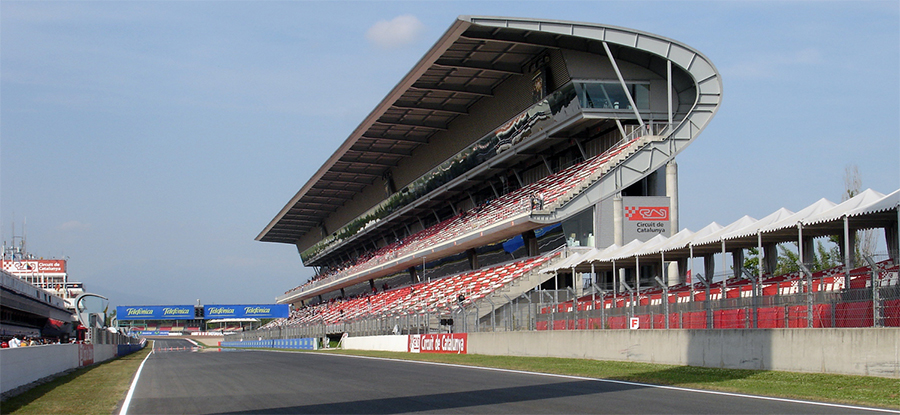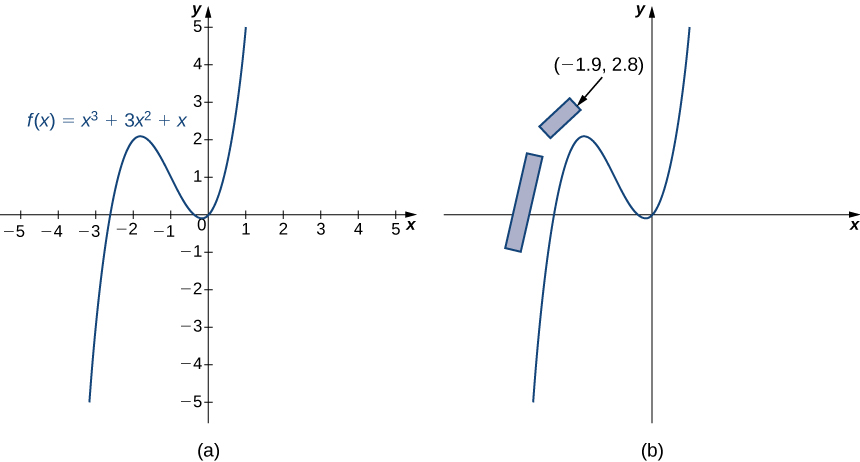# 3.3 Differentiation rules  (Page 6/14)

 Page 6 / 14

## Finding a velocity

The position of an object on a coordinate axis at time $t$ is given by $s\left(t\right)=\frac{t}{{t}^{2}+1}.$ What is the initial velocity of the object?

Since the initial velocity is $v\left(0\right)={s}^{\prime }\left(0\right),$ begin by finding ${s}^{\prime }\left(t\right)$ by applying the quotient rule:

${s}^{\prime }\left(t\right)=\frac{1\left({t}^{2}+1\right)-2t\left(t\right)}{{\left({t}^{2}+1\right)}^{2}}=\frac{1-{t}^{2}}{{\left({t}^{2}+1\right)}^{2}}.$

After evaluating, we see that $v\left(0\right)=1.$

Find the values of $x$ for which the line tangent to the graph of $f\left(x\right)=4{x}^{2}-3x+2$ has a tangent line parallel to the line $y=2x+3.$

$\frac{5}{8}$

## Formula one grandstands

Formula One car races can be very exciting to watch and attract a lot of spectators. Formula One track designers have to ensure sufficient grandstand space is available around the track to accommodate these viewers. However, car racing can be dangerous, and safety considerations are paramount. The grandstands must be placed where spectators will not be in danger should a driver lose control of a car ( [link] ).The grandstand next to a straightaway of the Circuit de Barcelona-Catalunya race track, located where the spectators are not in danger. **********

Safety is especially a concern on turns. If a driver does not slow down enough before entering the turn, the car may slide off the racetrack. Normally, this just results in a wider turn, which slows the driver down. But if the driver loses control completely, the car may fly off the track entirely, on a path tangent to the curve of the racetrack.

Suppose you are designing a new Formula One track. One section of the track can be modeled by the function $f\left(x\right)={x}^{3}+3x+x$ ( [link] ). The current plan calls for grandstands to be built along the first straightaway and around a portion of the first curve. The plans call for the front corner of the grandstand to be located at the point $\left(-1.9,2.8\right).$ We want to determine whether this location puts the spectators in danger if a driver loses control of the car.(a) One section of the racetrack can be modeled by the function f ( x ) = x 3 + 3 x + x . (b) The front corner of the grandstand is located at ( −1.9 , 2.8 ) .
1. Physicists have determined that drivers are most likely to lose control of their cars as they are coming into a turn, at the point where the slope of the tangent line is 1. Find the $\left(x,y\right)$ coordinates of this point near the turn.
2. Find the equation of the tangent line to the curve at this point.
3. To determine whether the spectators are in danger in this scenario, find the x -coordinate of the point where the tangent line crosses the line $y=2.8.$ Is this point safely to the right of the grandstand? Or are the spectators in danger?
4. What if a driver loses control earlier than the physicists project? Suppose a driver loses control at the point $\left(-2.5,0.625\right).$ What is the slope of the tangent line at this point?
5. If a driver loses control as described in part 4, are the spectators safe?
6. Should you proceed with the current design for the grandstand, or should the grandstands be moved?

## Key concepts

• The derivative of a constant function is zero.
• The derivative of a power function is a function in which the power on $x$ becomes the coefficient of the term and the power on $x$ in the derivative decreases by 1.
• The derivative of a constant c multiplied by a function f is the same as the constant multiplied by the derivative.
• The derivative of the sum of a function f and a function g is the same as the sum of the derivative of f and the derivative of g.
• The derivative of the difference of a function f and a function g is the same as the difference of the derivative of f and the derivative of g.
• The derivative of a product of two functions is the derivative of the first function times the second function plus the derivative of the second function times the first function.
• The derivative of the quotient of two functions is the derivative of the first function times the second function minus the derivative of the second function times the first function, all divided by the square of the second function.
• We used the limit definition of the derivative to develop formulas that allow us to find derivatives without resorting to the definition of the derivative. These formulas can be used singly or in combination with each other.

any genius online ? I need help!!
Pina
need to learn polynomial
Zakariya
i will teach...
nandu
I'm waiting
Zakariya
evaluate the following computation (x³-8/x-2)
teach me how to solve the first law of calculus.
what is differentiation
f(x) = x-2 g(x) = 3x + 5 fog(x)? f(x)/g(x)
fog(x)= f(g(x)) = x-2 = 3x+5-2 = 3x+3 f(x)/g(x)= x-2/3x+5
diron
pweding paturo nsa calculus?
jimmy
how to use fundamental theorem to solve exponential
find the bounded area of the parabola y^2=4x and y=16x
what is absolute value means?
Chicken nuggets
Hugh
🐔
MM
🐔🦃 nuggets
MM
(mathematics) For a complex number a+bi, the principal square root of the sum of the squares of its real and imaginary parts, √a2+b2 . Denoted by | |. The absolute value |x| of a real number x is √x2 , which is equal to x if x is non-negative, and −x if x is negative.
Ismael
find integration of loge x
find the volume of a solid about the y-axis, x=0, x=1, y=0, y=7+x^3
how does this work
Can calculus give the answers as same as other methods give in basic classes while solving the numericals?
log tan (x/4+x/2)
Rohan
Rohan
y=(x^2 + 3x).(eipix)
Claudia
Ismael
A Function F(X)=Sinx+cosx is odd or even?
neither
David
Neither
Lovuyiso
f(x)=1/1+x^2 |=[-3,1]
apa itu?
fauzi
determine the area of the region enclosed by x²+y=1,2x-y+4=0
Hi
MP
Hi too
Vic
hello please anyone with calculus PDF should share
Which kind of pdf do you want bro?
Aftab
hi
Abdul
can I get calculus in pdf
Abdul
explain for me
Usman
okay I have such documents
Fitzgerald
Hamza

#### Get Jobilize Job Search Mobile App in your pocket Now!By Donyea SweetsBy OpenStaxBy Dindin SecretoBy John GabrieliBy OpenStaxBy Tony PizurBy OpenStaxBy Hannah ShethBy OpenStaxBy Kevin Amaratunga